edHelper subscribers - Create a new printable

Sample edHelper.com - Algebra Worksheet

 Name _____________________________Date ___________________
The Law of Sines
Use the law of sines to solve for the unknown.  Assume that all of the angles are acute.

1.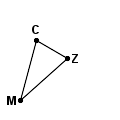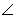M = 33MZ
=  21ZC
=  12C = ______2.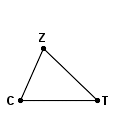Z = 70CZ
=  35.6CT
=  48.1C = ______3.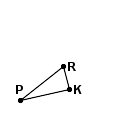K = 88R = 66PR
=  6.1PK
=  ______
4.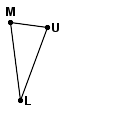U = 78M = 75UM
=  74LM
=  ______
5.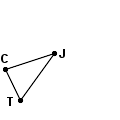C = 82TJ
=  29JC
=  26J = ______6.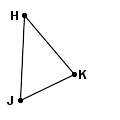K = 76J = 61JK
=  12KH
=  ______
7.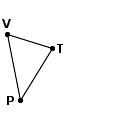T = 76PV
=  40.2TV
=  28.2V = ______8.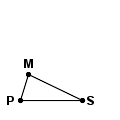S = 26P = 72SM
=  20PM
=  ______
9.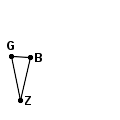B = 80Z = 25ZB
=  44BG
=  ______

Sample
This is only a sample worksheet.

edHelper subscribers - Create a new printable